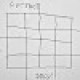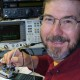# Arduino vs. Significant Figures: Preliminary 64-bit Fixed Point Exercise

Although it’s not advertised, the Arduino / AVR compiler mostly does the right thing with `long long` = `uint64_t` variables: add & subtract work fine, but multiplication & division discard anything that doesn’t fit into 64 bits. Fitting a 32 bit integer and a 32 bit fraction into such a thing should eliminate (most) problems with significant figures.

The general idea is to set up a `struct` giving access to the two 32 bit halves for direct manipulation, then overlay / `union` them with a single 64 bit integer for arithmetic purposes:

```struct ll_s {
uint32_t low;
uint32_t high;
};

union ll_u {
uint64_t ll_64;
struct ll_s ll_32;
};
```

Of course, the integer part still falls one bit shy of holding 2³². At the cost of one bit’s worth of resolution, you can still compute 2³² / 125×10⁶ by pre-dividing each quantity by 2:

```2^63 = [80000000 00000000]
2^63 / 125/2 M = [00000022 5c17d04d]
```

The low-order digit should be 0xe, not 0xd, but I think that’s survivable.

Unfortunately, `printf` doesn’t handle 64 bit quantities, necessitating some awkward conversion routines. “Printing” to a string seems the least awful method, as I’ll eventually squirt the strings to a display, not send them to the serial port:

```void PrintFractionLL(char *pBuffer,uint64_t *pLL) {
uint64_t Fraction;

Fraction = (uint32_t)*pLL;                      // copy 32 fraction bits, high order = 0
Fraction *= ONEGIG;                             // times 10^9 for conversion
Fraction >>= 32;                                // align integer part in low long
sprintf(pBuffer,"%09lu",(uint32_t)Fraction);    // convert low long to decimal
}

void PrintIntegerLL(char *pBuffer,uint64_t *pLL) {
sprintf(pBuffer,"%lu",*((uint32_t *)pLL+1));
}

void PrintDecimalLL(char *pBuffer,uint64_t *pLL) {
PrintIntegerLL(pBuffer,pLL);
pBuffer += strlen(pBuffer);       // pointer to end of integer part
*pBuffer++ = '.';                 // drop in the decimal point, tick pointer
PrintFractionLL(pBuffer,pLL);
}
```

The result seems nearly indistinguishable from the Right Answer:

```Integer:  34
Fraction: 359738367
Decimal: 34.359738367
```

This whole mess has a bunch of rough edges, but it looks promising. The code coalesced while fiddling around, so the `union` notation didn’t get much love at first.

The Arduino source code as a GitHub Gist:

 Long long integer exercise Ed Nisley - KE4ZNU - May 2017 Long long size = 8 bytes .. value = [12345678 9abcdef0] divided result = [01234567 89abcdef] 2^32 = [00000001 00000000] 125M = [00000000 07735940] 2^32 / 125M = [00000000 00000022] Scaled fixed point tests 2^63 = [80000000 00000000] 2^63 / 125/2 M = [00000022 5c17d04d] Integer: 34 Fraction: 359738367 Decimal: 34.359738367 Hz Per Ct: 0.029103830 60000: 60000.000000000 60 kHz as count: 2061584.302070550 0.1 Hz as count: 3.435973836 60000.1 Hz as count: 2061587.738044387 60000.1 Hz from count: 60000.078519806 60000.2 Hz as count: 2061591.174018223 60000.2 Hz from count: 60000.194935128 60000.2 Hz rnd count: 60000.224038958 Union size: 8 TestFreq: [0000ea60 395a9e00] Union ll: [0000ea60 395a9e00] From union: 60000.224038958 Buffer length: 15 Trunc dec: 60000.224
view raw LongLong.txt hosted with ❤ by GitHub
 // Long long integer exercise for 60 kHz crystal tester //-- Helper routine for printf() int s_putc(char c, FILE *t) { Serial.write(c); } char Buffer[10+1+10]; // string buffer for long long conversions #define ONEGIG 1000000000LL uint64_t CtPerHz; // will be 2^32 / 125 MHz uint64_t HzPerCt; // will be 125 MHz / 2^32 uint64_t TenthHzCt; // 0.1 Hz in counts struct ll_s { uint32_t low; uint32_t high; }; union ll_u { uint64_t ll_64; struct ll_s ll_32; }; //----------- // Long long print-to-buffer helpers // Assumes little-Endian layout void PrintHexLL(char *pBuffer,uint64_t *pLL) { sprintf(pBuffer,"%08lx %08lx",*((uint32_t *)pLL+1),(uint32_t)*pLL); } // converts 9 decimal digits void PrintFractionLL(char *pBuffer,uint64_t *pLL) { uint64_t Fraction; Fraction = (uint32_t)*pLL; // copy 32 fraction bits, high order = 0 Fraction *= ONEGIG; // times 10^9 for conversion Fraction >>= 32; // align integer part in low long sprintf(pBuffer,"%09lu",(uint32_t)Fraction); // convert low long to decimal } void PrintIntegerLL(char *pBuffer,uint64_t *pLL) { sprintf(pBuffer,"%lu",*((uint32_t *)pLL+1)); } void PrintDecimalLL(char *pBuffer,uint64_t *pLL) { PrintIntegerLL(pBuffer,pLL); pBuffer += strlen(pBuffer); // pointer to end of integer part *pBuffer++ = '.'; // drop in the decimal point, tick pointer PrintFractionLL(pBuffer,pLL); } //----------- void setup () { Serial.begin (115200); fdevopen(&s_putc,0); // set up serial output for printf() Serial.println ("Long long integer exercise"); Serial.println ("Ed Nisley - KE4ZNU - May 2017"); unsigned long long LongLong; uint64_t LongLong2; LongLong = 0x123456789abcdef0LL; printf("Long long size = %d bytes\n",sizeof(LongLong)); printf(" .. value = [%08lx %08lx]\n",(long)(LongLong >> 32),(long)(LongLong & 0x00000000ffffffffLL)); LongLong /= 16; printf(" divided result = [%08lx %08lx]\n",(long)(LongLong >> 32),(long)LongLong); LongLong = 1LL << 32; printf(" 2^32 = [%08lx %08lx]\n",(long)(LongLong >> 32),(long)LongLong); LongLong2 = 125000000LL; printf(" 125M = [%08lx %08lx]\n",(long)(LongLong2 >> 32),(long)LongLong2); LongLong /= LongLong2; printf("2^32 / 125M = [%08lx %08lx]\n",(long)(LongLong >> 32),(long)LongLong); Serial.println("Scaled fixed point tests"); uint64_t TestFreq,TestCount; CtPerHz = 1LL << 63; // start with 2^31 to avoid overflow PrintHexLL(Buffer,&CtPerHz); printf("2^63 = [%s]\n",Buffer); CtPerHz /= 125000000LL / 2; // divided by 2 to to match 2^31 PrintHexLL(Buffer,&CtPerHz); printf("2^63 / 125/2 M = [%s]\n",Buffer); PrintIntegerLL(Buffer,&CtPerHz); printf("Integer: %s\n",Buffer); PrintFractionLL(Buffer,&CtPerHz); printf("Fraction: %s\n",Buffer); PrintDecimalLL(Buffer,&CtPerHz); printf("Decimal: %s\n",Buffer); HzPerCt = 125000000LL; // 125 MHz / 2^32, directly to fraction part PrintDecimalLL(Buffer,&HzPerCt); printf("Hz Per Ct: %s\n",Buffer); TestFreq = 60000LL << 32; PrintDecimalLL(Buffer,&TestFreq); printf("60000: %s\n",Buffer); TestCount = 60000LL * CtPerHz; PrintDecimalLL(Buffer,&TestCount); printf("60 kHz as count: %s\n",Buffer); TenthHzCt = CtPerHz / 10; // 0.1 Hz as counts PrintDecimalLL(Buffer,&TenthHzCt); printf("0.1 Hz as count: %s\n",Buffer); TestCount += TenthHzCt; PrintDecimalLL(Buffer,&TestCount); printf("60000.1 Hz as count: %s\n",Buffer); TestFreq = (TestCount >> 32) * HzPerCt; PrintDecimalLL(Buffer,&TestFreq); printf("60000.1 Hz from count: %s\n",Buffer); TestCount = (60000LL * CtPerHz) + (2 * TenthHzCt); PrintDecimalLL(Buffer,&TestCount); printf("60000.2 Hz as count: %s\n",Buffer); TestFreq = (TestCount >> 32) * HzPerCt; PrintDecimalLL(Buffer,&TestFreq); printf("60000.2 Hz from count: %s\n",Buffer); TestFreq = ((TestCount + (TenthHzCt / 2)) >> 32) * HzPerCt; PrintDecimalLL(Buffer,&TestFreq); printf("60000.2 Hz rnd count: %s\n",Buffer); union ll_u LongLongUnion, TempLLU; printf("Union size: %d\n",sizeof(LongLongUnion)); LongLongUnion.ll_64 = TestFreq; PrintHexLL(Buffer,&TestFreq); printf("TestFreq: [%s]\n",Buffer); PrintHexLL(Buffer,&LongLongUnion.ll_64); printf("Union ll: [%s]\n",Buffer); TempLLU.ll_64 = LongLongUnion.ll_32.low; TempLLU.ll_64 *= ONEGIG; // times 10^9 for conversion TempLLU.ll_64 >>= 32; // align integer part in low long sprintf(Buffer,"%lu.%09lu",LongLongUnion.ll_32.high,TempLLU.ll_32.low); printf("From union: %s\n",Buffer); sprintf(Buffer,"%lu.%09lu",LongLongUnion.ll_32.high,TempLLU.ll_32.low); printf("Buffer length: %d\n",strlen(Buffer)); Buffer[strlen(Buffer) - 9 + 3] = 0; printf(" Trunc dec: %s\n",Buffer); } //----------- void loop () { }
view raw LongLongTest.ino hosted with ❤ by GitHub

## 3 thoughts on “Arduino vs. Significant Figures: Preliminary 64-bit Fixed Point Exercise”

1.William Rutiser says:

What tool chain do use for Arduino work?
You have inspired me to at least find and dump out the contents of a box of Arduino stuff from Dec 2010.

1.Ed says:

Not much of a chain: the plain old Arduino IDE set to “use external editor” on the landscape monitor and KDE fullscreen on the portrait monitor. Save the file, click upload, and away it goes.

The bone-stock IDE lets me get away with columns / article minus all the verbiage explaining how to squirt programs into microcontrollers.